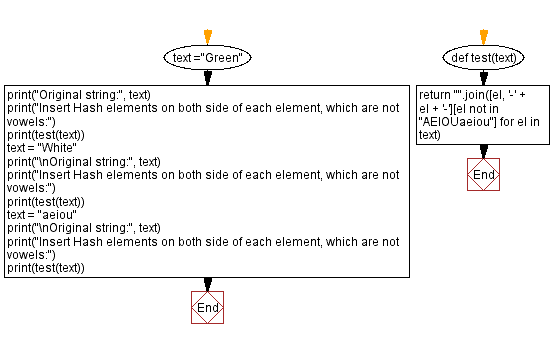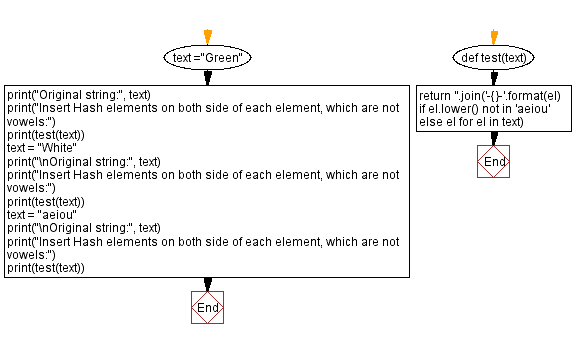﻿ Python - Hash elements# Python Exercises: Hash elements

## Python String: Exercise-108 with Solution

Write a Python program to that takes a string and returns # on both sides each element, which are not vowels.

Sample Data:
("Green" -> "-G--r-ee-n-"
("White") -> "-W--h-i-t-e"
(“aeiou") -> "aeiou"

Sample Solution-1:

Python Code:

``````def test(text):
return "".join([el, '-' + el + '-'][el not in "AEIOUaeiou"] for el in text)
text ="Green"
print("Original string:", text)
print("Insert Hash elements on both side of each element, which are not vowels:")
print(test(text))
text = "White"
print("\nOriginal string:", text)
print("Insert Hash elements on both side of each element, which are not vowels:")
print(test(text))
text = "aeiou"
print("\nOriginal string:", text)
print("Insert Hash elements on both side of each element, which are not vowels:")
print(test(text))
```
```

Sample Output:

```Original string: Green
Insert Hash elements on both side of each element, which are not vowels:
-G--r-ee-n-

Original string: White
Insert Hash elements on both side of each element, which are not vowels:
-W--h-i-t-e

Original string: aeiou
Insert Hash elements on both side of each element, which are not vowels:
aeiou
```

Flowchart:## Visualize Python code execution:

The following tool visualize what the computer is doing step-by-step as it executes the said program:

Sample Solution-2:

Python Code:

``````def test(text):
return ''.join('-{}-'.format(el) if el.lower() not in 'aeiou' else el for el in text)
text ="Green"
print("Original string:", text)
print("Insert Hash elements on both side of each element, which are not vowels:")
print(test(text))
text = "White"
print("\nOriginal string:", text)
print("Insert Hash elements on both side of each element, which are not vowels:")
print(test(text))
text = "aeiou"
print("\nOriginal string:", text)
print("Insert Hash elements on both side of each element, which are not vowels:")
print(test(text))
```
```

Sample Output:

```Original string: Green
Insert Hash elements on both side of each element, which are not vowels:
-G--r-ee-n-

Original string: White
Insert Hash elements on both side of each element, which are not vowels:
-W--h-i-t-e

Original string: aeiou
Insert Hash elements on both side of each element, which are not vowels:
aeiou
```

Flowchart:## Visualize Python code execution:

The following tool visualize what the computer is doing step-by-step as it executes the said program:

Python Code Editor:

Have another way to solve this solution? Contribute your code (and comments) through Disqus.

Previous Python Exercise: Two strings contain three letters at the same index.
Next Python Exercise: Count the number of leap years within the range.

What is the difficulty level of this exercise?

Test your Programming skills with w3resource's quiz.

﻿

## Python: Tips of the Day

Getting sorted iterable (can sort by "compare" function):

```>>> a = [1, 2, -3]
>>> sorted(a)
[-3, 1, 2]

>>> sorted(a,key=abs)
[1, 2, -3]
```